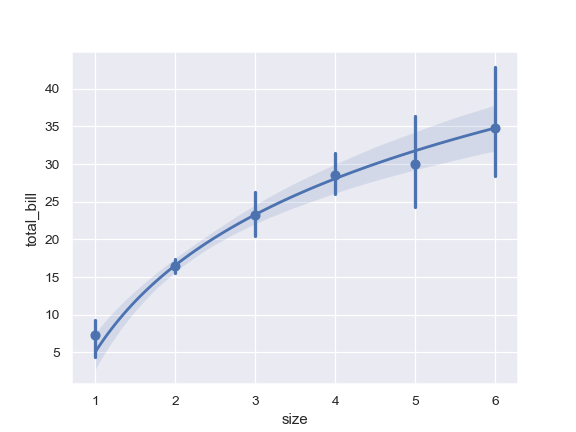# seaborn.regplot¶

`seaborn.``regplot`(x, y, data=None, x_estimator=None, x_bins=None, x_ci='ci', scatter=True, fit_reg=True, ci=95, n_boot=1000, units=None, seed=None, order=1, logistic=False, lowess=False, robust=False, logx=False, x_partial=None, y_partial=None, truncate=True, dropna=True, x_jitter=None, y_jitter=None, label=None, color=None, marker='o', scatter_kws=None, line_kws=None, ax=None)

Plot data and a linear regression model fit.

There are a number of mutually exclusive options for estimating the regression model. See the tutorial for more information.

Parameters
x, y: string, series, or vector array

Input variables. If strings, these should correspond with column names in `data`. When pandas objects are used, axes will be labeled with the series name.

Tidy (“long-form”) dataframe where each column is a variable and each row is an observation.

x_estimatorcallable that maps vector -> scalar, optional

Apply this function to each unique value of `x` and plot the resulting estimate. This is useful when `x` is a discrete variable. If `x_ci` is given, this estimate will be bootstrapped and a confidence interval will be drawn.

x_binsint or vector, optional

Bin the `x` variable into discrete bins and then estimate the central tendency and a confidence interval. This binning only influences how the scatterplot is drawn; the regression is still fit to the original data. This parameter is interpreted either as the number of evenly-sized (not necessary spaced) bins or the positions of the bin centers. When this parameter is used, it implies that the default of `x_estimator` is `numpy.mean`.

x_ci“ci”, “sd”, int in [0, 100] or None, optional

Size of the confidence interval used when plotting a central tendency for discrete values of `x`. If `"ci"`, defer to the value of the `ci` parameter. If `"sd"`, skip bootstrapping and show the standard deviation of the observations in each bin.

scatterbool, optional

If `True`, draw a scatterplot with the underlying observations (or the `x_estimator` values).

fit_regbool, optional

If `True`, estimate and plot a regression model relating the `x` and `y` variables.

ciint in [0, 100] or None, optional

Size of the confidence interval for the regression estimate. This will be drawn using translucent bands around the regression line. The confidence interval is estimated using a bootstrap; for large datasets, it may be advisable to avoid that computation by setting this parameter to None.

n_bootint, optional

Number of bootstrap resamples used to estimate the `ci`. The default value attempts to balance time and stability; you may want to increase this value for “final” versions of plots.

unitsvariable name in `data`, optional

If the `x` and `y` observations are nested within sampling units, those can be specified here. This will be taken into account when computing the confidence intervals by performing a multilevel bootstrap that resamples both units and observations (within unit). This does not otherwise influence how the regression is estimated or drawn.

seedint, numpy.random.Generator, or numpy.random.RandomState, optional

Seed or random number generator for reproducible bootstrapping.

orderint, optional

If `order` is greater than 1, use `numpy.polyfit` to estimate a polynomial regression.

logisticbool, optional

If `True`, assume that `y` is a binary variable and use `statsmodels` to estimate a logistic regression model. Note that this is substantially more computationally intensive than linear regression, so you may wish to decrease the number of bootstrap resamples (`n_boot`) or set `ci` to None.

lowessbool, optional

If `True`, use `statsmodels` to estimate a nonparametric lowess model (locally weighted linear regression). Note that confidence intervals cannot currently be drawn for this kind of model.

robustbool, optional

If `True`, use `statsmodels` to estimate a robust regression. This will de-weight outliers. Note that this is substantially more computationally intensive than standard linear regression, so you may wish to decrease the number of bootstrap resamples (`n_boot`) or set `ci` to None.

logxbool, optional

If `True`, estimate a linear regression of the form y ~ log(x), but plot the scatterplot and regression model in the input space. Note that `x` must be positive for this to work.

{x,y}_partialstrings in `data` or matrices

Confounding variables to regress out of the `x` or `y` variables before plotting.

truncatebool, optional

If `True`, the regression line is bounded by the data limits. If `False`, it extends to the `x` axis limits.

{x,y}_jitterfloats, optional

Add uniform random noise of this size to either the `x` or `y` variables. The noise is added to a copy of the data after fitting the regression, and only influences the look of the scatterplot. This can be helpful when plotting variables that take discrete values.

labelstring

Label to apply to either the scatterplot or regression line (if `scatter` is `False`) for use in a legend.

colormatplotlib color

Color to apply to all plot elements; will be superseded by colors passed in `scatter_kws` or `line_kws`.

markermatplotlib marker code

Marker to use for the scatterplot glyphs.

{scatter,line}_kwsdictionaries

Additional keyword arguments to pass to `plt.scatter` and `plt.plot`.

axmatplotlib Axes, optional

Axes object to draw the plot onto, otherwise uses the current Axes.

Returns
axmatplotlib Axes

The Axes object containing the plot.

`lmplot`

Combine `regplot()` and `FacetGrid` to plot multiple linear relationships in a dataset.

`jointplot`

Combine `regplot()` and `JointGrid` (when used with `kind="reg"`).

`pairplot`

Combine `regplot()` and `PairGrid` (when used with `kind="reg"`).

`residplot`

Plot the residuals of a linear regression model.

Notes

The `regplot()` and `lmplot()` functions are closely related, but the former is an axes-level function while the latter is a figure-level function that combines `regplot()` and `FacetGrid`.

It’s also easy to combine combine `regplot()` and `JointGrid` or `PairGrid` through the `jointplot()` and `pairplot()` functions, although these do not directly accept all of `regplot()`’s parameters.

Examples

Plot the relationship between two variables in a DataFrame:

```>>> import seaborn as sns; sns.set(color_codes=True)
>>> ax = sns.regplot(x="total_bill", y="tip", data=tips)
```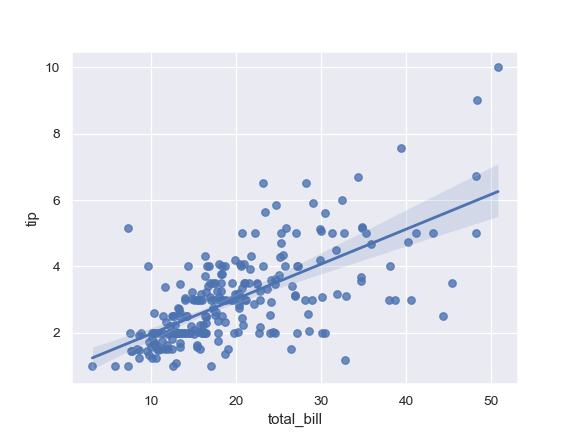Plot with two variables defined as numpy arrays; use a different color:

```>>> import numpy as np; np.random.seed(8)
>>> mean, cov = [4, 6], [(1.5, .7), (.7, 1)]
>>> x, y = np.random.multivariate_normal(mean, cov, 80).T
>>> ax = sns.regplot(x=x, y=y, color="g")
```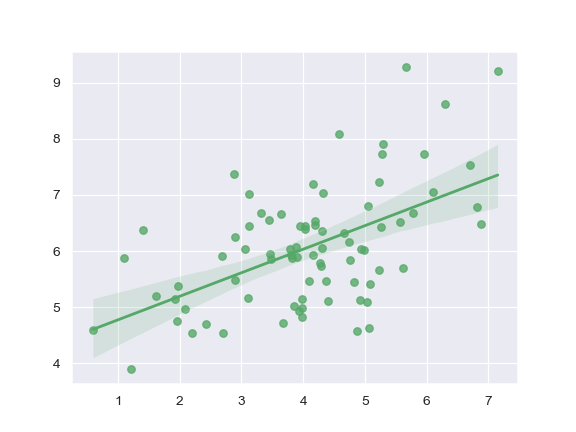Plot with two variables defined as pandas Series; use a different marker:

```>>> import pandas as pd
>>> x, y = pd.Series(x, name="x_var"), pd.Series(y, name="y_var")
>>> ax = sns.regplot(x=x, y=y, marker="+")
```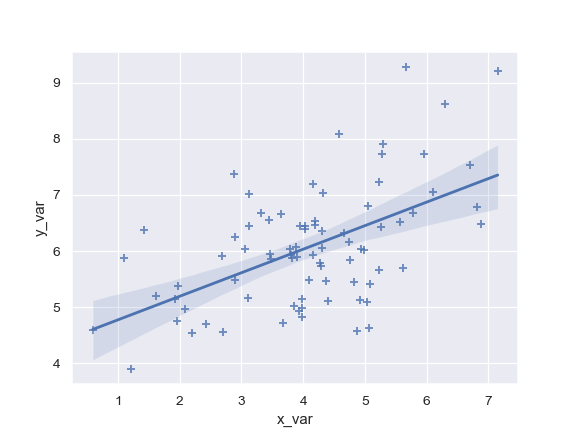Use a 68% confidence interval, which corresponds with the standard error of the estimate, and extend the regression line to the axis limits:

```>>> ax = sns.regplot(x=x, y=y, ci=68, truncate=False)
```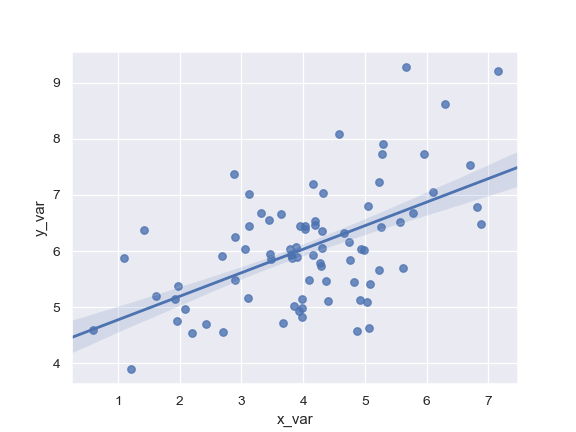Plot with a discrete `x` variable and add some jitter:

```>>> ax = sns.regplot(x="size", y="total_bill", data=tips, x_jitter=.1)
```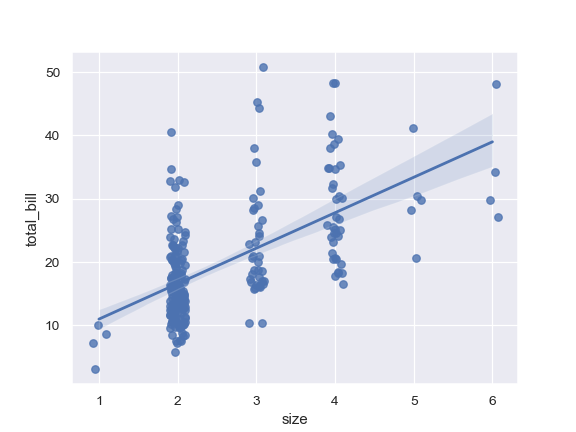Plot with a discrete `x` variable showing means and confidence intervals for unique values:

```>>> ax = sns.regplot(x="size", y="total_bill", data=tips,
...                  x_estimator=np.mean)
```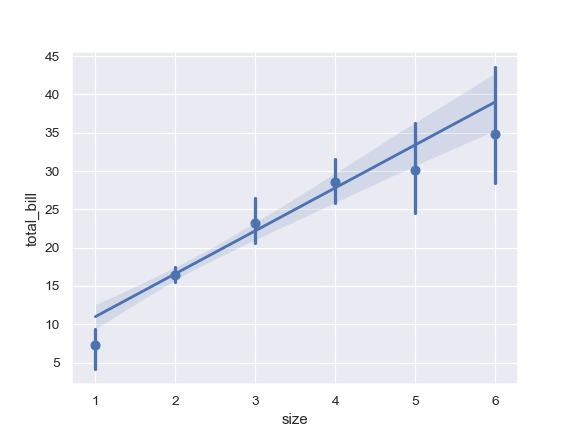Plot with a continuous variable divided into discrete bins:

```>>> ax = sns.regplot(x=x, y=y, x_bins=4)
```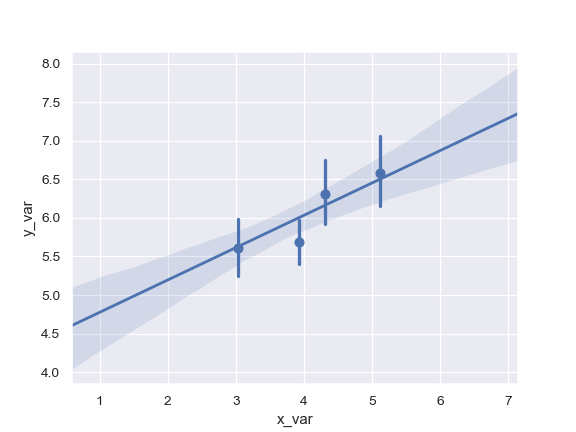Fit a higher-order polynomial regression:

```>>> ans = sns.load_dataset("anscombe")
>>> ax = sns.regplot(x="x", y="y", data=ans.loc[ans.dataset == "II"],
...                  scatter_kws={"s": 80},
...                  order=2, ci=None)
```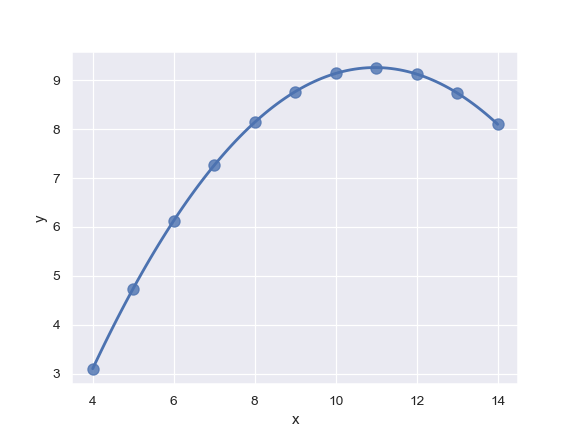Fit a robust regression and don’t plot a confidence interval:

```>>> ax = sns.regplot(x="x", y="y", data=ans.loc[ans.dataset == "III"],
...                  scatter_kws={"s": 80},
...                  robust=True, ci=None)
```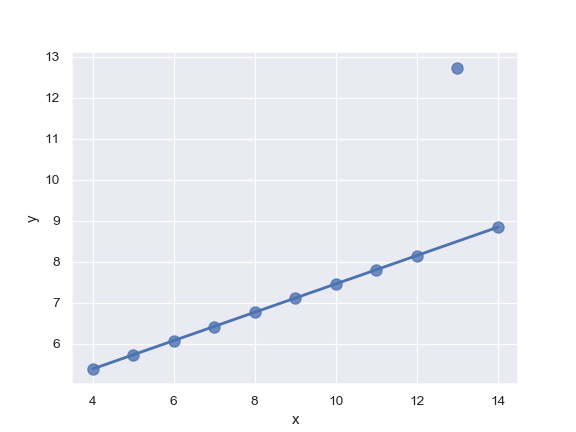Fit a logistic regression; jitter the y variable and use fewer bootstrap iterations:

```>>> tips["big_tip"] = (tips.tip / tips.total_bill) > .175
>>> ax = sns.regplot(x="total_bill", y="big_tip", data=tips,
...                  logistic=True, n_boot=500, y_jitter=.03)
```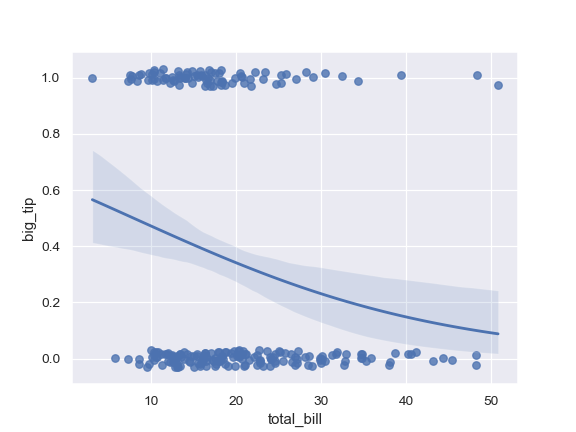Fit the regression model using log(x):

```>>> ax = sns.regplot(x="size", y="total_bill", data=tips,
...                  x_estimator=np.mean, logx=True)
```